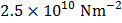# When a wire of length 10m is subjected to a force of 100 N along its length, the lateral strain produced ism. The Poisson’s ratio was found to be 0.4. If the area of cross-section of wire is, its Young’s modulus is a)b)c)d)## Question ID - 55998 :- When a wire of length 10m is subjected to a force of 100 N along its length, the lateral strain produced ism. The Poisson’s ratio was found to be 0.4. If the area of cross-section of wire is, its Young’s modulus is a)b)c)d)3537

 (a) Poisson’s ratioorYoung’s modulusIfis the origin andare the tangents from the origin to the circle, then circumcenter of the triangleis a)b)c)d)